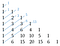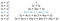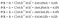# Top 10 Secrets of Pascal’s Triangle

## Binomial Theorem, Fibonacci Sequence, Sierpinski Triangle & More

The beauty of Pascal’s Triangle is that it’s so simple, yet so mathematically rich. It’s one of those novelties in math that highlight just how extraordinary this logical system we’ve devised truly is.

So let’s do this!

Top 10 things you probably didn’t know were hiding in Pascal’s Triangle!!

# But First…How to Build Pascal’s Triangle

• At the top center of your paper write the number “1.”
• On the next row write two 1’s, forming a triangle.
• On each subsequent row start and end with 1’s and compute each interior term by summing the two numbers above it.

# Secret #1: Hidden Sequences

Note: I’ve left-justified the triangle to help us see these hidden sequences.

The first two columns aren’t too interesting, they’re just the ones and the natural numbers.

The next column is the triangular numbers. You can think of the triangular numbers as the number of dots it takes to make various sized triangles.

Similarly the fourth column is the tetrahedral numbers, or triangular pyramidal numbers. As their name suggests they represent the number of dots needed to make pyramids with triangle bases.

The columns continue in this way, describing the “simplices” which are just extrapolations of this triangle/tetrahedron idea to arbitrary dimensions. The next column is the 5-simplex numbers, followed by the 6-simplex numbers and so on.

# Secret #2: Powers of Two

If we sum each row, we obtain powers of base 2, beginning with 2⁰=1.

# Secret #3: Powers of Eleven

The triangle also reveals powers of base 11. All you have to do is squish the numbers in each row together. Which is easy enough for the first 5 rows, but what about when we get to double-digit entries?

Turns out all you have to do is carry the tens place over to the number on its left.Demonstrating how to carry the ten’s place in row six to yield 11⁵

# Secret #4: Perfect Squares

We can locate the perfect squares of the natural numbers in column 2 by summing the number to the right with the number below the number to the right.

For example,

• 2² → 1+3=4
• 3² → 3+6
• 4² → 6+10=16

and so on…

# Secret #5: The Fibonacci Sequence

To uncover the hidden Fibonacci Sequence sum the diagonals of the left-justified Pascal Triangle.The first 7 numbers in Fibonacci’s Sequence: 1, 1, 2, 3, 5, 8, 13, … found in Pascal’s Triangle

# Secret #6: The Sierpinski Triangle

Using the original orientation of Pascal’s Triangle, shade in all the odd numbers and you’ll get a picture that looks similar to the famous fractal Sierpinski Triangle.

# Secret #7: Combinatorics

Perhaps the most interesting relationship found in Pascal’s Triangle is how we can use it to find the combinatorial numbers.

Recall the combinatorics formula n choose k (if you’re blanking on what I’m talking about check out this post for a review). We find that in each row of Pascal’s Triangle n is the row number and k is the entry in that row, when counting from zero.

So if you want to calculate 4 choose 2 look at the 5th row, 3rd entry (since we’re counting from zero) and you’ll find the answer is 6.

# Secret #8: Expanding Binomials

Suppose you have the binomial (x + y) and you want to raise it to a power such as 2 or 3. Normally you’d need to go through the long process of multiplying, but with Pascal’s Triangle you can avoid the hassle and skip to the answer!

For example, let’s expand (x+y)³. Since we’re raising (x+y) to the 3rd power, use the values in the fourth row of Pascal’s as the coefficients of your expansion. Then fill in the x and y terms as outlined below.Note: Each term’s degree totals to the power that (x+y) is being raised to.

# Secret #9: Binomial Theorem

Multiplying powers of (x+y) is cool, but how often do we come across the need to solve that exact problem? Probably, not too often. Wouldn’t it be handy if we could generalize the idea from the last section into a more usable form?

Well, turns out that’s the Binomial Theorem:

Don’t let the notation scare you. It’s similar to what we did in the last section. The best way to understand any formula is to work an example.

Let’s try (2x–3)³.

Let x from our formula be the first term and y be the second. Then x=2x, y=–3, n=3 and k is the integers from 0 to n=3, in this case k={0, 1, 2, 3}.

First I’ll fill in the formula using all the above values except k:

It still looks a little strange, but we’re getting closer.

Next fill in the values for k. Recall that k has 4 values, so we need to fill out 4 different versions and add them together.

Simplify terms with exponents of zero and one:

Compute the rest of the exponent terms:

We already know that the combinatorial numbers come from Pascal’s Triangle, so we can simply look up the 4th row and substitute in the values 1, 3, 3, 1 respectively:

Finish multiplying and simplifying:

With the Binomial Theorem you can raise any binomial to any power without the hassle of actually multiplying out the terms — making this a seriously handy tool!

For a step-by-step walk through of how to do a binomial expansion with Pascal’s Triangle, check out my tutorial ⬇️

# Secret #10: Binomial Distribution

The Binomial Distribution describes a probability distribution based on experiments that have two possible outcomes. The most classic example of this is tossing a coin.

Say we’re interested in tossing heads, we’ll call this a “success” with probability p. Then tossing tails is the “failure” case and has the complement probability 1–p.

If we design an experiment with 3 trials (aka coin tosses) and want to know the likelihood of tossing heads, we can use the probability mass function (pmf) for the binomial distribution, where n is the number of trials and k is the number of successes, to find the distribution of probabilities.

Hey, that looks familiar! It’s almost the same formula as we used above in the Binomial Theorem except there’s no summation and instead of x’s and y’s we have p’s and 1–p’s.

Assuming a success probability of 0.5 (p=0.5), let’s calculate the chance of flipping heads zero, one, two, or three times.

Fill in the equation for n=3 and k=0, 1, 2, 3 and complete the computations:Use the combinatorial numbers from Pascal’s Triangle: 1, 3, 3, 1

The likelihood of flipping zero or three heads are both 12.5%, while flipping one or two heads are both 37.5%.

Whew!

Top 10 secrets of Pascal’s Triangle, what a blast! So I’m curious: which ones did you know and which were new to you?

## ❤ STAY CONNECTED ❤

Stay up-to-date with everything Math Hacks is up to!

Written by

Written by

## Brett Berry

#### Check out my YouTube channel “Math Hacks” for hands-on math tutorials and lots of math love ♥️